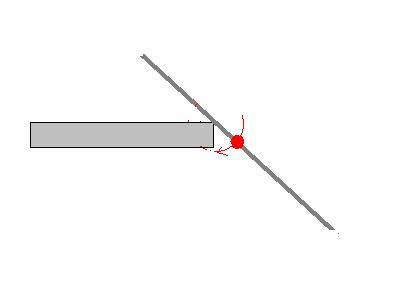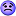# Coefficient of friction; rod slipping off edge of table

• Nathanael
In summary, the coefficient of friction is \mu and the rod should slip if the acceleration of the center of mass exceeds μF N m .

Homework Helper

## Homework Statement

A rod of mass M and length L is placed horizontally on a table such that the center of mass is a distance L/6 from the edge of the table (CoM is hanging off the table). The rod is released from rest and slips after reaching an angle θ. Find the coefficient of friction $\mu$

2. The attempt at a solution
Friction is the only horizontal external force acting, so once the horizontal acceleration of the center of mass exceeds $\frac{\mu F_N}{m}$ the rod should slip.

Call the horizontal and vertical accelerations of the CoM $a_x$ and $a_y$ respectively. The normal force can be found from the relationship $mg-F_n=ma_y$ therefore the key constraint for the problem is:

$\mu(mg-ma_y)=ma_x$
or
$\mu(g-a_y)=a_x$
...
$a_x=\frac{L}{6}ω^2\cos(\theta)+\frac{L}{6}α\sin(\theta)$
$a_y=\frac{L}{6}α\cos(\theta)-\frac{L}{6}ω^2\sin(\theta)$
...
$α=mg\frac{L}{6}\cos(\theta)$
$ω^2=\frac{2}{I}mg\frac{L}{6}\sin(\theta)$
...
$I=\frac{mL^2}{9}$

Sorry I don't have time at the moment to show all the intermediate algebraic steps or to explain each equation, but putting it all together I get

$\mu=\frac{\cos(\theta)\sin(\theta)}{2-\cos^2(\theta)}$

Whereas the correct answer is supposed to be proportional to $\tan(\theta)$

Are any of my equations wrong?

Nathanael said:
Friction is the only horizontal external force acting
I hate to say, "Skip the math and think qualitatively about what happens," but this is one of those problems where it might help up front. As the rod begins to fall, the COM moves toward the table.

Bystander said:
As the rod begins to fall, the COM moves toward the table.
Yes, isn't it the friction that allows this?

Nathanael said:
Yes, isn't it the friction that allows this?
Nathanael said:
once the horizontal acceleration of the center of mass exceeds μF N m \frac{\mu F_N}{m} the rod should slip.
Static friction. The rod is pivoting about that point. The normal force is changing direction as the rod falls, and changing magnitude as the unsupported end accelerates.

•Nathanael
Bystander said:
Static friction.
Yeah I should have mentioned, every time I spoke of friction or $\mu$ I was talking about static friction.

Bystander said:
The normal force is changing direction as the rod falls
Thank you, I did not realize this. I was thinking it would always be vertical and only decrease in magnitude.

Also I think I forgot to mention, I'm measuring θ as the angle the rod makes with the horizontal.

So then, I guess the direction of the normal force would always be perpendicular to the rod?
And my equation $mg-F_n=ma_y$ would become $mg-F_n\cos(\theta)=ma_y$

So I have one last question. When considering the maximum force that friction can apply, would I use only the component of the normal force perpendicular to the table?

Last edited:
Nathanael said:
always be perpendicular to the rod?
Would be the way I'd play it.
Nathanael said:
only the component of the normal force perpendicular to the table?
This is where I get a little "fuzzy." If it's a "perfect edge" on the table, there is only line or point contact with the rod (don't know what shape its cross-section is) but that doesn't affect the friction, and I'd stick with the "normal" being perpendicular to the rod at that line or point contact.

Before the rod starts to slip, its centre of mass moves along a circle. Think of centripetal force - what are the radial force components?
You need the speed of the CoM for the centripetal force, but energy is conserved before the slipping occurs.
The rod is pivoted about the edge of the table as soon as the piece on the table raises. The normal force is perpendicular to the rod.ehild said:
Before the rod starts to slip, its centre of mass moves along a circle. Think of centripetal force - what are the radial force components?
That is how I got these equations:
Nathanael said:
$a_x=\frac{L}{6}ω^2\cos(\theta)+\frac{L}{6}α\sin(\theta)$
$a_y=\frac{L}{6}α\cos(\theta)-\frac{L}{6}ω^2\sin(\theta)$
(Well actually I did it two ways, one way from centripetal and tangential components of acceleration and another way from the chain/product rules, but both methods agree.)

ehild said:
You need the speed of the CoM for the centripetal force, but energy is conserved before the slipping occurs.
That is how I get this equation:
Nathanael said:
$ω^2=\frac{2}{I}mg\frac{L}{6}\sin(\theta)$
(Well again I did it another way out of curiosity but it agreed with CoE.)

ehild said:
The rod is pivoted about the edge of the table as soon as the piece on the table raises. The normal force is perpendicular to the rod.
I did not know normal force was perpendicular to the rod until bystander brought it up, but using this information I still get the wrong answer. I get:

$(\mu-\tan\theta)(g-a_y)=a_x$

$\mu=\tan\theta+\frac{\cos\theta\sin\theta}{2-\cos^2\theta}=\frac{2\tan\theta}{2-\cos^2\theta}$

Nathanael said:
I did not know normal force was perpendicular to the rod until bystander brought it up, but using this information I still get the wrong answer. I get:

$(\mu-\tan\theta)(g-a_y)=a_x$

How did you get it?

ehild said:
How did you get it?
We can get a "value" for the normal force from the vertical net force equation:
(force of gravity minus vertical component of normal force equals vertical acceleration*mass)
$mg-F_n\cos(\theta)=ma_y$
$F_n=\frac{1}{\cos\theta}(mg-ma_y)$

Then we write an equation for the horizontal net force:
(force of friction minus horizontal component of normal force equals horizontal acceleration*mass)
$\mu F_n\cos\theta-F_n\sin(\theta)=ma_x$
$\mu (mg-ma_y)-\tan\theta(mg-ma_y)=ma_x$
$(\mu-\tan\theta)(g-a_y)=a_x$

Nathanael said:
We can get a "value" for the normal force from the vertical net force equation:
(force of gravity minus vertical component of normal force equals vertical acceleration*mass)
$mg-F_n\cos(\theta)=ma_y$
$F_n=\frac{1}{\cos\theta}(mg-ma_y)$
You ignored the vertical component of the friction.

Your method is utterly complicated. It would be much simpler in polar coordinates.

ehild said:
You ignored the vertical component of the friction.
I was thinking it was only horizontal. So if the force of friction acts parallel to the rod, would I use the entire normal force to find the maximum frictional force?

Nathanael said:
if the force of friction acts parallel to the rod
If it slips, the direction of movement will be along the line of the rod. Therefore friction acts along that line.
Nathanael said:
would I use the entire normal force to find the maximum frictional force?
Yes.

•Nathanael
haruspex said:
If it slips, the direction of movement will be along the line of the rod. Therefore friction acts along that line.
That's a nice way to think about it, thanks.

ehild said:
Your method is utterly complicated. It would be much simpler in polar coordinates.
It was a bit complicated, but it gave the correct answer. (I'm surprised I made no algebra mistakes!) Now that I've correctly solved it from that perspective, I am curious about how you would solve it in polar coordinates. I don't see how to do it, but I don't think I've ever solved any problem in polar coordinates...

This is a video how you get the acceleration in plane planar coordinates.

.

## \vec a = (\ddot {r} -r \omega^2 ) \hat{ r} + (2 \omega \dot r+r \dot \omega) \hat {\theta}##

In this problem, the radius is constant.The acceleration has a radial component ar = -rω2, and a tangential component, along the circle: aθ = r dω/dt.
You resolve gravity into radial (parallel to the rod ) and normal components and collect the radial components : Fs-mgsin(θ) which is equal to the centripetal force, and the normal components mgcos (θ) -FN, equal to the tangential force. Friction opposes the relative motion of the contact surfaces, and at the edge of the table the slipping motion would be along the rod: radial.

Last edited:
•Nathanael
ehild said:
It would be much simpler in polar coordinates.
I wrote the two equations for ar and aθ

$mg\sin\theta+\mu F_N=ma_r=-mrω^2=-m\frac{L}{6}ω^2$

$mg\cos\theta-F_N=ma_θ=mr\frac{dω}{dt}=m\frac{L}{6}α$

This gives me an answer of μ=-2tanθ
It's almost right... the answer from the first method was μ=tanθ

It was much quicker, but why'd it turn out negative?

The force of friction is opposite to the force along the slope. -μFN+mgsinθ=-mrω2.

ehild said:
The force of friction is opposite to the force along the slope. -μFN+mgsinθ=-mrω2.
Oops. But then I get (2/3)tanθI considered FN the magnitude of the normal force.
Nathanael said:
Oops. But then I get (2/3)tanθHow do you do it? I get 2tan(theta)

ehild said:
I get 2tan(theta)
Ah, I do too. Sorry about that.

I am going to make an effort to use polar coordinates more often (or at least to consider whether they might be easier!)

Thanks ehild

Nathanael said:
I am going to make an effort to use polar coordinates more often (or at least to consider whether they might be easier!)

Whenever you solve problems concerning circular motion and use centripetal force, you work in polar coordinates :)

And I succeeded to get the same result with your method at last. It was just terrible to get itLast edited: